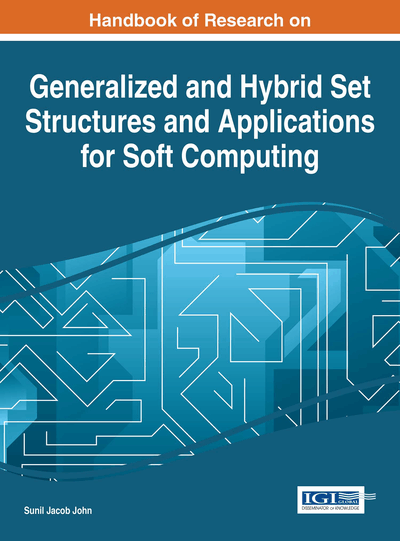Refined Neutrosophic Sets and Refined Neutrosophic Soft Sets: Theory and Applications

Irfan Deli (Kilis 7 Aralık University, Turkey)
DOI: 10.4018/978-1-4666-9798-0.ch016

Abstract

Refined neutrosophic sets (RNS) are a generalization of a neutrosophic sets, intuitionistic fuzzy sets, fuzzy sets, intuitionistic fuzzy multi-sets and fuzzy multi-sets. Similarly, refined neutrosophic soft sets (RNSS) are a generalization of a neutrosophic soft sets, intuitionistic fuzzy soft sets, fuzzy soft sets, intuitionistic fuzzy soft multi-sets and fuzzy soft multi-sets. These sets are a powerful general formal framework that has been proposed to present uncertainty, imprecise, incomplete, inaccurate and inconsistent information which exist in real life. This chapter will survey concept of RNS and concept of RNSS with basic definitions and will present an efficient approach for both RNS and RNSS. Also, the chapter will introduce an application of RNS in medical diagnosis problem, pattern recognition and an application of RNSS in decision making to illustrate the advantage of the proposed approach.
Chapter Preview
Top

1. Background

Many theories have been introduced to present uncertainty, imprecise, incomplete, indeterminacy, inaccurate and inconsistent information which exist in real life such as; fuzzy set theory by Zadeh (1965), intuitionistic fuzzy set theory by Atanassov (1986), soft set theory by Molodtsov (1999), neutrosophic set theory by Smarandache (1998), and so on. After the introduction of these theories, many scholars have done a lot of good researches in this filed such as; Broumi et al. (2014a, 2014b, 2014c), Çağman and Deli (2012a, 2012b), Deli et al. (2014a), Deli and Çağman (2014b), Deli andBroumi (2015a, 2015b). After Yager (1986) originally presented a new theory, is called theory multi-set the concept of multi-set as useful structures arising in much area of mathematics and computer sciences, therefore, many researchers from time to time developed a number of generalization of multi set theory by using above theories. For example; on fuzzy multisets (e.g. Muthuraj & Balamurugan 2013; Sebastian, & Ramakrishnan 2010, 2011a, 2011b; Syropoulos 2010,2012), on intuitionistic fuzzy multisets (e.g. Ejegwa & Awolola 2014), Rajarajeswari and Uma (2013a, 2013b, 2013c, 2014a, 2014b), Shinoj and John (2012)), on soft multisets (e.g. Alkhazaleh and Salleh Hassan (2011), Babitha and John (2013), Balami and Ibrahim (2013), NeogSut(2012)), on vague soft multisets (e.g. Alhazaymeh and Hassan (2014)), on fuzzy soft multisets (e.g. Alkhazaleh and Salleh (2012)), on intuitionistic fuzzy soft multisets (e.g. Mukherjee et al. (2013), Mukherjee and Das (2014)).

Key Terms in this Chapter

Refined Neutrosophic Logic: The refined logic is generalization ofthe classical neutrosophic logic to n-valued neutrosophic logic, by extending each neutrosophic component T, I and F into T 1 , T 2 ,…,T m , I 1 , I 2 ,…,I n and F 1 , F 2 ,…,F r , respectively.

Standard Part and Non-Standard: In a neutrosophic set, each neutrosophic component T, I and F is an element of [ - 0, 1 + ]. In here, 1 + = 1+?, where 1 is its standard part and ? its non-standard part. Similarly, - 0= 0-?, where 0 is its standard part and ? its non-standard part.

Multiset: Multiset is a generalization of the concept of a set. For example; { a , a , a , b , b , b,b }

Complete Chapter List

Search this Book:
Reset